# 7.5: Vector Projection

•• Contributed by CK12
• CK12

Projecting one vector onto another explicitly answers the question: “how much of one vector goes in the direction of the other vector?”  The dot product is useful because it produces a scalar quantity that helps to answer this question.  In this concept, you will produce an actual vector not just a scalar.

Why is vector projection useful when considering pulling a box in the direction of $$v$$ instead of horizontally in the direction of $$u ?$$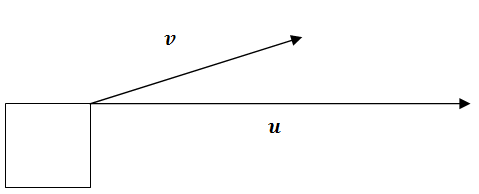### Projections

Consider the question from above.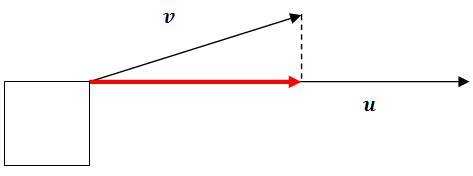The definition of vector projection for the indicated red vector is the called $$\operatorname{proj}_{u} v$$. When you read proju $$v$$ you should say "the vector projection of $$v$$ onto $$u$$." This implies that the new vector is going in the direction of $$u$$. The vector projection is the vector produced when one vector is resolved into two component vectors, one that is parallel to the second vector and one that is perpendicular to the second vector. The parallel vector is the vector projection. Conceptually, this means that if someone was pulling the box at an angle and strength of vector $$v$$ then some of their energy would be wastedpulling the box up and some of the energy would actually contribute to pulling the box horizontally.

The definition of scalar projection is simply the length of the vector projection. When the scalar projection is positive it means that the angle between the two vectors is less than $$90^{\circ}$$. When the scalar projection is negative it means that the two vectors are heading in opposite directions.

The vector projection formula can be written two ways.  The version on the left is most simplified, but the version on the right makes the most sense conceptually. A.

$$p r o j_{u} v=\left(\frac{v \cdot u}{|u|^{2}}\right) u=\left(\frac{v \cdot u}{|u|}\right) \frac{u}{|u|}$$

The proof of the vector projection formula is as follows:

Given two vectors $$u, v,$$ what is $$p r o j_{u} v ?$$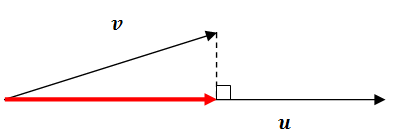First note that the projected vector in red will go in the direction of $$u$$. This means that it will be a product of the unit vector $$\frac{u}{|u|}$$ and the length of the red vector (the scalar projection). In order to find the scalar projection, note the right triangle, the unknown angle $$\theta$$ between the two vectors and the cosine ratio.

$$\cos \theta=\frac{\text {scalar projection}}{|v|}$$

Recall that $$\cos \theta=\frac{u \cdot v}{|u||v|}$$. Now just substitute and simplify to find the length of the scalar projection.

\begin{aligned} \cos \theta &=\frac{\text {scalar projection}}{|v|} \\ \frac{u \cdot v}{|u||v|} &=\frac{\text {scalar projection}}{|v|} \\ \frac{u \cdot v}{|u|} &=\text {scalar projection} \end{aligned}

Now you have the length of the vector projection and the direction you want it to go:

$$p r o j_{u} v=\left(\frac{u \cdot v}{|u|}\right) \frac{u}{|u|}$$

### Examples

Example 1

Earlier, you were asked why vector projection useful when considering pulling a box in the direction of instead of horizontally in the direction of $$u$$. Vector projection is useful in physics applications involving force and work.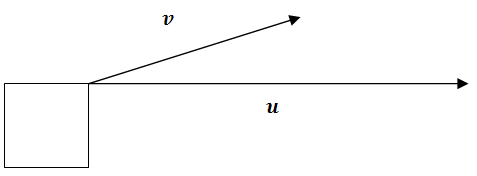When the box is pulled by vector $$v$$ some of the force is wasted pulling up against gravity. In real life this may be useful because of friction, but for now this energy is inefficiently wasted in the horizontal movement of the box.

Example 2

Find the scalar projection of vector $$v=<3,4>$$ onto vector $$u=<5,-12>$$.

As noted earlier, the scalar projection is the magnitude of the vector projection. This was shown to be $$\left(\frac{u \cdot v}{|u|}\right)$$ where $$u$$ is the vector being projected onto.

$$\frac{u \cdot v}{|u|}=\frac{<5,-12>\cdot<3,4>}{13}=\frac{15-48}{13}=-\frac{33}{13}$$

Example 3

Find the vector projection of vector $$v=<3,4>$$ onto vector $$u=<5,-12>$$

Since the scalar projection has already been found in Example 2 , you should multiply the scalar by the "onto" unit vector.

$$-\frac{33}{13}<\frac{5}{13},-\frac{12}{13}>=<-\frac{165}{169}, \frac{396}{169}>$$

Example 4

Sketch the vector $$<-2,-2>$$ and $$<4,-2>$$. Explain using a sketch why a negative scalar projection of $$<-2,-2>$$ onto $$<4,-2>$$ makes sense.

First plot the two vectors and extend the "onto" vector. When the vector projection occurs, the vector $$<-2,2>$$ goes in the opposite direction of the vector $$<4,-2>$$. This will create a vector projection going in the opposite direction of $$<4,-2>$$.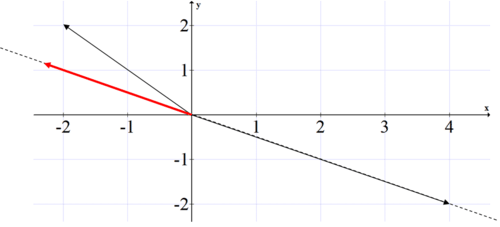Example 5

A father is pulling his daughter up a hill. The hill has a $$20^{\circ}$$ incline. The daughter is on a sled that sits on the ground and has a rope that the father pulls with a force of 100 Ib as he walks. The rope makes a $$39^{\circ}$$ angle with the slope. What is the effective force that the father exerts moving his daughter and the sled up the hill?

The box represents the girl and the sled. The blue arrow indicates the father's 100 Ib force. Notice that the question asks for simply the amount of force which means scalar projection. since this is not dependent on the slope of this hill, we can rotate our perspective and still get the same scalar projection.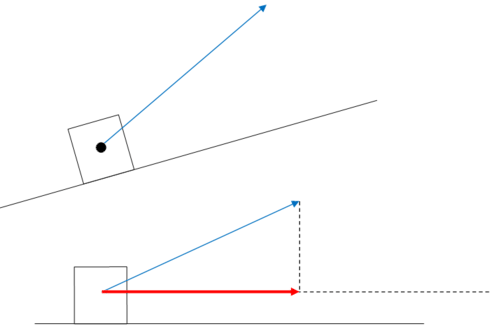The components of the father's force vector is $$100<\cos 39^{\circ}, \sin 39^{\circ}>$$ and the "onto" vector is any vector horizontally to the right. since we are only looking for the length of the horizontal component and you already have the angle between the two vectors, the scalar projection is:

$$100 \cdot \cos 39^{\circ} \approx 77.1 l b$$

Review

1. Sketch vectors $$<2,4>$$ and $$<2,1>$$.

2. What is the vector projection of $$<2,4>$$ onto $$<2,1>?$$ Sketch the projection.

3. Sketch vectors $$<-2,1>$$ and $$<-1,3>$$.

4. What is the vector projection of $$<-1,3>$$ onto $$<-2,1>?$$ Sketch the projection.

5. Sketch vectors $$<6,2>$$ and $$<8,1>$$.

6. What is the vector projection of $$<6,2>$$ onto $$<8,1>$$ ? Sketch the projection.

7. Sketch vectors $$<1,7>$$ and $$<6,3>$$.

8. What is the vector projection of $$<1,7>$$ onto $$<6,3>?$$ Sketch the projection.

9. A box is on the side of a hill inclined at $$30^{\circ}$$. The weight of the box is 40 pounds. What is the magnitude of the force required to  keep the box from sliding down the hill?

10. Sarah is on a sled on the side of a hill inclined at $$60^{\circ}$$. The weight of Sarah and the sled is 125 pounds. What is the magnitude of the force required for Sam to keep Sarah from sliding down the hill.

11. $$A 1780$$ pound car is parked on a street that makes an angle of $$15^{\circ}$$ with the horizontal. Find the magnitude of the force required to keep the car from rolling down the hill.

12. $$\mathrm{A} 1900$$ pound car is parked on a street that makes an angle of $$10^{\circ}$$ with the horizontal. Find the magnitude of the force required to keep the car from rolling down the hill.

13. $$\mathrm{A} 30$$ pound force that makes an angle of $$32^{\circ}$$ with an inclined plane is pulling a box up the plane. The inclined plane makes a $$20^{\circ}$$ angle with the horizontal. What is the magnitude of the effective force pulling the box up the plane?

14. $$\mathrm{A} 22$$ pound force that makes an angle of $$12^{\circ}$$ with an inclined plane is pulling a box up the plane. The inclined plane makes a $$25^{\circ}$$ angle with the horizontal. What is the magnitude of the effective force pulling the box up the plane?

15. Anne pulls a wagon on a horizontal surface with a force of 50 pounds. The handle of the wagon makes an angle of $$30^{\circ}$$ with the ground. What is the magnitude of the effective force pulling the wagon?